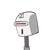# The sum of squares of two consecutive odd natural number is394.Find the number​

The sum of squares of two consecutive odd natural number is394.Find the number​

### 1 thought on “The sum of squares of two consecutive odd natural number is394.Find the number​”

1.The two consecutive numbers are 13 and 15

Step-by-step explanation:

Consider one number be (2n−1), then the next consecutive odd number will be (2n+1)

⇒(2n−1)²+(2n+1)² =394

⇒4n²+1−4n+4n²+1+4n=394

⇒8n²+2=394

⇒8n²=392

⇒n² =49

⇒n=7

Therefore, the two consecutive numbers are

(2n−1)=14−1=13

(2n+1)=14+1=15

Hence, the two consecutive numbers are 13 and 15

Hope it helps